# I look for RSI Expert32

Hi ,

have someone an RSI Expert with buy over 70, and sell down the 30 line.

maybe with stops and takeprofit.

thank you very much

wz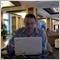Moderator
117007

May be this one.

Files:
rsi.mq4 6 kb32

great

thats wonderfull...

thank you very very much

best forum of the world :-)1177

Hello,

I note that Mr Newdigital became a professional of the software Gordago software. Cheer

webzukunft:
thats wonderfull...

thank you very very much

best forum of the world :-)

Now that you have this EA, will be able you to have the kindness to give the results like your comments. Thank you webzukunft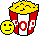77

https://www.mql5.com/en/forum/general

that's the best RSI expert around here128

Rsi ea

Hi

Can somebody help me in writing a RSI EA , RSI value is 13 and the RSI MA values is 5 , cross of the RSI MA will trigger Buy or Sell as the case may be and it should be a auto trade in metatrader 4.

Unimak128

where RSI 5 is the moving average and RSI 13 is the normal RSI. Break or cross over on the up side triggers a buy and on the downside triggers a sell .

I need a EA which will do auto trade with the above condition built in.

Files:
rsi.jpg 45 kb128

One more Pic for easy reference. Pip difference should be 3 pips between bid and ask .

Files:
rsi_-ma.jpg 566 kb128

Hi

I got the EA done for a website and compiled and saw that there is no errors but when i put it on the chart it does not trigger any trade although the allow live trading is ticked in the options box. Can somebody tell me where i went wrong

================================================

//+------------------------------------------------------------------+

//| This MQL is generated by Expert Advisor Builder |

//| |

//| In no event will author be liable for any damages whatsoever. |

//| Use at your own risk. |

//| |

//+------------------- DO NOT REMOVE THIS HEADER --------------------+

#define SIGNAL_NONE 0

#define SIGNAL_SELL 2

#define SIGNAL_CLOSESELL 4

extern int MagicNumber = 0;

extern bool SignalMail = False;

extern bool EachTickMode = True;

extern double Lots = 0.01;

extern int Slippage = 3;

extern bool UseStopLoss = True;

extern int StopLoss = 3;

extern bool UseTakeProfit = True;

extern int TakeProfit = 6;

extern bool UseTrailingStop = True;

extern int TrailingStop = 3;

int BarCount;

int Current;

bool TickCheck = False;

//+------------------------------------------------------------------+

//| expert initialization function |

//+------------------------------------------------------------------+

int init() {

BarCount = Bars;

if (EachTickMode) Current = 0; else Current = 1;

return(0);

}

//+------------------------------------------------------------------+

//| expert deinitialization function |

//+------------------------------------------------------------------+

int deinit() {

return(0);

}

//+------------------------------------------------------------------+

//| expert start function |

//+------------------------------------------------------------------+

int start() {

int Order = SIGNAL_NONE;

int Total, Ticket;

double StopLossLevel, TakeProfitLevel;

if (EachTickMode && Bars != BarCount) TickCheck = False;

Total = OrdersTotal();

Order = SIGNAL_NONE;

//+------------------------------------------------------------------+

//| Variable Begin |

//+------------------------------------------------------------------+

double Var1 = iRSI(NULL, 0, 13, PRICE_CLOSE, Current + 0);

double Var2 = iRSI(NULL, 0, 5, PRICE_CLOSE, Current + 0);

double Buy1_1 = iRSI(NULL, 0, 13, PRICE_CLOSE, Current + 0);

double Buy1_2 = iRSI(NULL, 0, 5, PRICE_CLOSE, Current + 0);

double Sell1_1 = iRSI(NULL, 0, 13, PRICE_CLOSE, Current + 0);

double Sell1_2 = iRSI(NULL, 0, 5, PRICE_CLOSE, Current + 0);

//+------------------------------------------------------------------+

//| Variable End |

//+------------------------------------------------------------------+

//Check position

for (int i = 0; i < Total; i ++) {

if(OrderType() <= OP_SELL && OrderSymbol() == Symbol()) {

//Close

//+------------------------------------------------------------------+

//+------------------------------------------------------------------+

//+------------------------------------------------------------------+

//+------------------------------------------------------------------+

if (Order == SIGNAL_CLOSEBUY && ((EachTickMode && !TickCheck) || (!EachTickMode && (Bars != BarCount)))) {

OrderClose(OrderTicket(), OrderLots(), Bid, Slippage, MediumSeaGreen);

if (SignalMail) SendMail("[Signal Alert]", "[" + Symbol() + "] " + DoubleToStr(Bid, Digits) + " Close Buy");

if (!EachTickMode) BarCount = Bars;

continue;

}

//Trailing stop

if(UseTrailingStop && TrailingStop > 0) {

if(Bid - OrderOpenPrice() > Point * TrailingStop) {

if(OrderStopLoss() < Bid - Point * TrailingStop) {

OrderModify(OrderTicket(), OrderOpenPrice(), Bid - Point * TrailingStop, OrderTakeProfit(), 0, MediumSeaGreen);

if (!EachTickMode) BarCount = Bars;

continue;

}

}

}

} else {

//Close

//+------------------------------------------------------------------+

//| Signal Begin(Exit Sell) |

//+------------------------------------------------------------------+

//+------------------------------------------------------------------+

//| Signal End(Exit Sell) |

//+------------------------------------------------------------------+

if (Order == SIGNAL_CLOSESELL && ((EachTickMode && !TickCheck) || (!EachTickMode && (Bars != BarCount)))) {

if (SignalMail) SendMail("[Signal Alert]", "[" + Symbol() + "] " + DoubleToStr(Ask, Digits) + " Close Sell");

if (!EachTickMode) BarCount = Bars;

continue;

}

//Trailing stop

if(UseTrailingStop && TrailingStop > 0) {

if((OrderOpenPrice() - Ask) > (Point * TrailingStop)) {

if((OrderStopLoss() > (Ask + Point * TrailingStop)) || (OrderStopLoss() == 0)) {

OrderModify(OrderTicket(), OrderOpenPrice(), Ask + Point * TrailingStop, OrderTakeProfit(), 0, DarkOrange);

if (!EachTickMode) BarCount = Bars;

continue;

}

}

}

}

}

}

//+------------------------------------------------------------------+

//| Signal Begin(Entry) |

//+------------------------------------------------------------------+

if (Sell1_1 < Sell1_2) Order = SIGNAL_SELL;

//+------------------------------------------------------------------+

//| Signal End |

//+------------------------------------------------------------------+

if (Order == SIGNAL_BUY && ((EachTickMode && !TickCheck) || (!EachTickMode && (Bars != BarCount)))) {

//Check free margin

if (AccountFreeMargin() < (1000 * Lots)) {

Print("We have no money. Free Margin = ", AccountFreeMargin());

return(0);

}

if (UseStopLoss) StopLossLevel = Ask - StopLoss * Point; else StopLossLevel = 0.0;

if (UseTakeProfit) TakeProfitLevel = Ask + TakeProfit * Point; else TakeProfitLevel = 0.0;

Ticket = OrderSend(Symbol(), OP_BUY, Lots, Ask, Slippage, StopLossLevel, TakeProfitLevel, "Buy(#" + MagicNumber + ")", MagicNumber, 0, DodgerBlue);

if(Ticket > 0) {

Print("BUY order opened : ", OrderOpenPrice());

if (SignalMail) SendMail("[Signal Alert]", "[" + Symbol() + "] " + DoubleToStr(Ask, Digits) + " Open Buy");

} else {

Print("Error opening BUY order : ", GetLastError());

}

}

if (EachTickMode) TickCheck = True;

if (!EachTickMode) BarCount = Bars;

return(0);

}

}

//Sell

if (Order == SIGNAL_SELL && ((EachTickMode && !TickCheck) || (!EachTickMode && (Bars != BarCount)))) {

//Check free margin

if (AccountFreeMargin() < (1000 * Lots)) {

Print("We have no money. Free Margin = ", AccountFreeMargin());

return(0);

}

if (UseStopLoss) StopLossLevel = Bid + StopLoss * Point; else StopLossLevel = 0.0;

if (UseTakeProfit) TakeProfitLevel = Bid - TakeProfit * Point; else TakeProfitLevel = 0.0;

Ticket = OrderSend(Symbol(), OP_SELL, Lots, Bid, Slippage, StopLossLevel, TakeProfitLevel, "Sell(#" + MagicNumber + ")", MagicNumber, 0, DeepPink);

if(Ticket > 0) {

Print("SELL order opened : ", OrderOpenPrice());

if (SignalMail) SendMail("[Signal Alert]", "[" + Symbol() + "] " + DoubleToStr(Bid, Digits) + " Open Sell");

} else {

Print("Error opening SELL order : ", GetLastError());

}

}

if (EachTickMode) TickCheck = True;

if (!EachTickMode) BarCount = Bars;

return(0);

}

}

if (!EachTickMode) BarCount = Bars;

return(0);

}

//+------------------------------------------------------------------+

========================================================3

Fixing RSI problem in EA

Hi,

I'm having a slight problem here, I've an EA works on RSI only, it should open a trade when RSI cross line30 from BELOW, but it opens now false trades when it's near line30.

So anybody here knows the right code for opening an order when it crosses from BELOW.... Also that order would be after how much bars and can't it be opened after it crosses line30 immediatly....

Thanks,

elbarck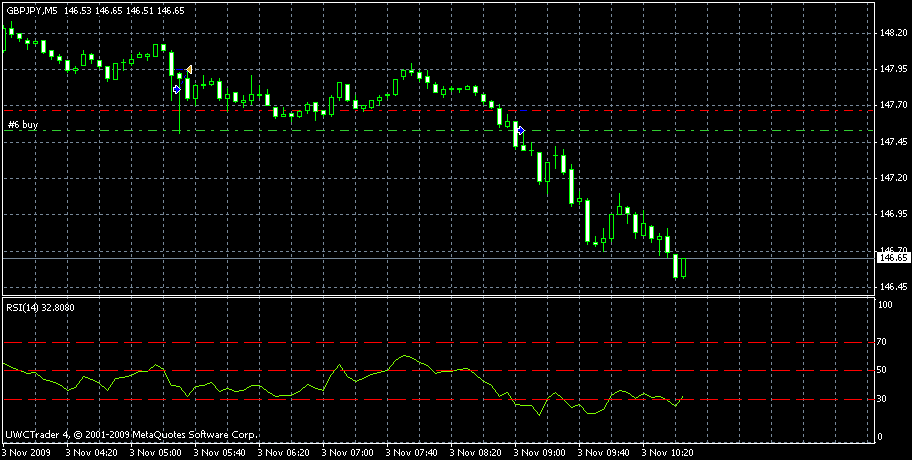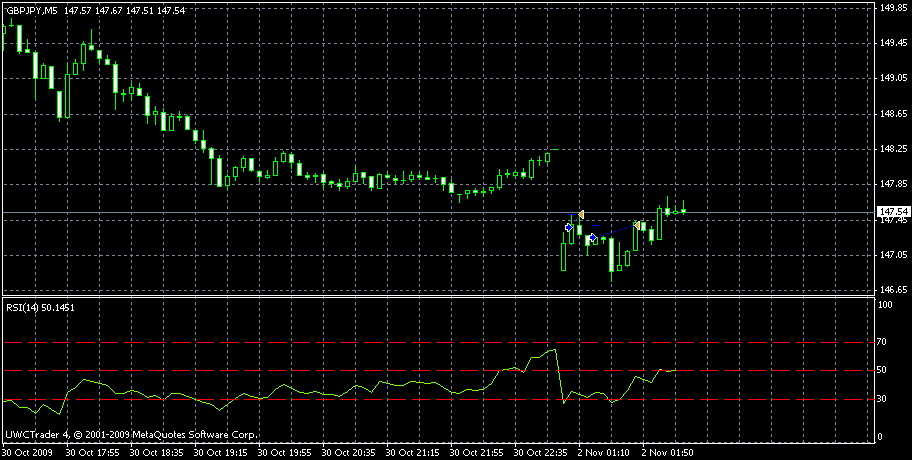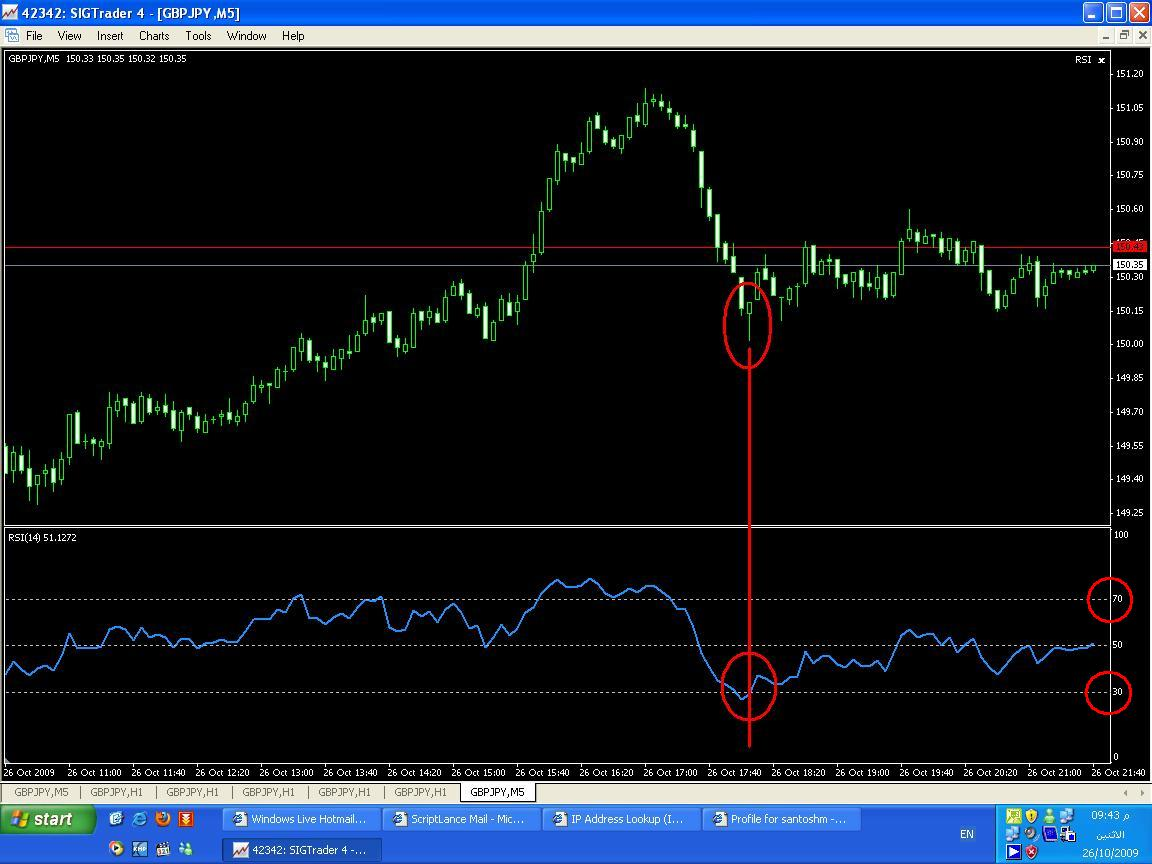3

RSI ea

Please I am trying to code this EA without success

buy only if RSI (period 18) is = 29 and latest 5 ticks values where <= 29

close the buy above only if (RSI period 18) is = 70

AND

sell only if RSI (period 18) is =71 and latest 5 ticks where => 71

close sell above only if RSI (period 18) is = 28.5

If possible limit buy open operations to max 20

If possible limit sell open operations to max 20

Anyone is able to code this ?